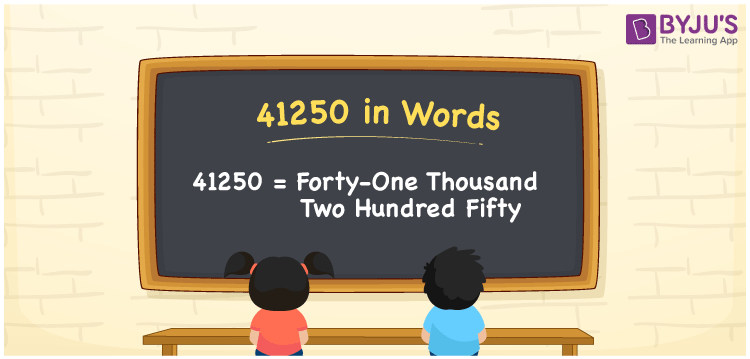# 41250 in Words

41250 in words is written as Forty-one thousand two hundred fifty. In both the International System of Numerals and the Indian System of Numerals, 41250 is written as Forty-one thousand two hundred fifty. The number 41250 is a Cardinal Number as it could represent some quantity. For example, “the course fee is 41250 rupees”.

 41250 in Words Forty-one thousand two hundred fifty Forty-one thousand two hundred fifty in Number 41250

## 41250 in English Words

41250 in English words is read as “Forty-one thousand two hundred fifty”.## How to Write 41250 in Words?

To write 41250 in words, we shall use the place value chart. In the place value chart, put 4 in the ten thousand, 1 in the thousands, 2 in the hundreds, 5 in the tens and 0 in the ones. Let us make a place value chart to write the number 41250 in words.

 Ten Thousands Thousands Hundreds Tens Ones 4 1 2 5 0

Thus, we can write the expanded form as

4 × Ten Thousand + 1 × Thousand + 2 × Hundred + 5 × Ten + 0 × One

= 4 × 10000 + 1 × 1000 + 2 × 100 + 5 × 10 + 0 × 1

= 40000 + 1000 + 200 + 50 + 0

= 41250

= Forty-one thousand two hundred fifty.

41250 is a natural number, the successor of 41249 and the predecessor of 41251.

41250 in words – Forty-one thousand two hundred fifty

• Is 41250 an odd number? – No
• Is 41250 an even number? – Yes
• Is 41250 a perfect square number? – No
• Is 41250 a perfect cube number? – No
• Is 41250 a prime number? – No
• Is 41250 a composite number? – Yes

## Frequently Asked Questions on 41250 in Words

Q1

### How to write 41250 in words?

41250 in words is written as Forty-one thousand two hundred fifty.
Q2

### How to write 41250 in the International and Indian System of Numerals?

In both, the system of numerals, 41250 in words, is written as Forty-one thousand two hundred fifty.
Q3

### How to write 41250 in a place value chart?

In the place value chart, write 4 in the ten thousand, 1 in the thousands, 2 in the hundreds, 5 in the tens and 0 in the ones, respectively.